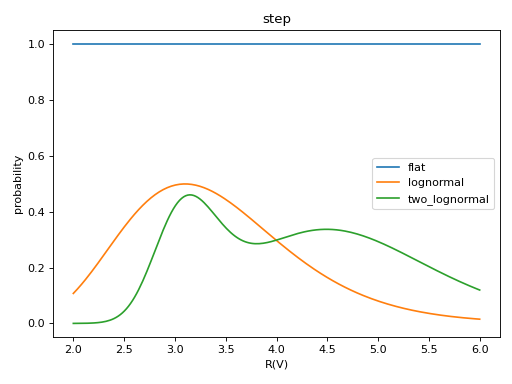# Priors¶

Priors on the parameters are set in the beast_settings.txt file using python dictionaries to give the prior model and any related parameters.

All priors are normalized to have an average value of 1. This is possible as the priors are relevant in a relative sense, not absolute and this avoids numerical issues with too large or small numbers.

New prior models can be added in the specific code regions by defining a new prior model name and creating the necessary code to support it. The code files of interest are beast/physicsmodel/prior_weights_[stars,dust].py. New models added should be documented here.

## Stellar¶

### Age¶

The age prior is the star formation rate (SFR) and can be

1. Flat in linear age

```age_prior_model = {'name': 'flat'}
```

or

```age_prior_model = {'name': 'flat_linear'}
```
1. Flat in log age

```age_prior_model = {'name': 'flat_log'}
```
1. Set by bins spaced in logage.

For example, step like priors can be specified by:

```age_prior_model = {'name': 'bins_histo',
'logages': [6.0, 7.0, 8.0, 9.0, 10.0],
'values': [1.0, 2.0, 1.0, 5.0, 3.0]}
```

Or using bin edges (where N = N_values+1) like those output by `np.histogram()`:

```age_prior_model = {'name': 'bins_histo',
'logages': [6.0, 7.0, 8.0, 9.0, 10.0],
'values': [1.0, 2.0, 1.0, 5.0]}
```

For example, lines connecting the bin value of the priors can be specified by:

```age_prior_model = {'name': 'bins_interp',
'logages': [6.0, 7.0, 8.0, 9.0, 10.0],
'values': [1.0, 2.0, 1.0, 5.0, 3.0]}
```

4. An exponentially decreasing SFR (in time, but here increasing with age) with a 0.1 Gyr time constant (with `tau` parameter in Gyr):

```age_prior_model = {'name': 'exp',
'tau': 0.1}
```

Plot showing examples of the possible age prior models with the parameters given above.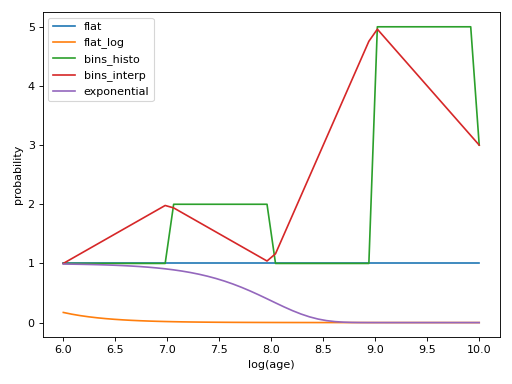### Mass¶

The mass prior is set by the choice of an Initial Mass Function (IMF). The two mass function supported are:

1. Kroupa (details needed)

```mass_prior_model = {'name': 'kroupa'}
```
1. Salpeter (details needed)

```mass_prior_model = {'name': 'salpeter'}
```

There is also a flat mass prior. This is useful for creating grids for BEAST verification (see Simulations), and should not be used for a standard fitting run.

```mass_prior_model = {'name': 'flat'}
```

Plot showing examples of the possible mass prior models with the parameters given above.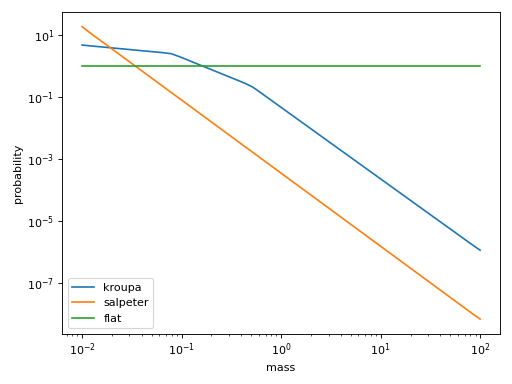### Metallicity¶

The metallicity prior can be

1. Flat

```met_prior_model = {'name': 'flat'}
```

Plot showing examples of the possible metallicity prior models with the parameters given above.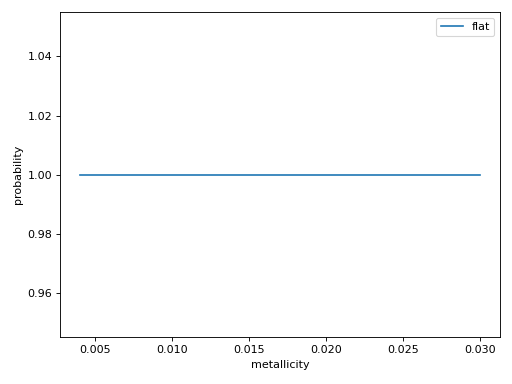### Distance¶

The distance prior can be

1. Flat

```distance_prior_model = {'name': 'flat'}
```

Plot showing examples of the possible distance prior models with the parameters given above.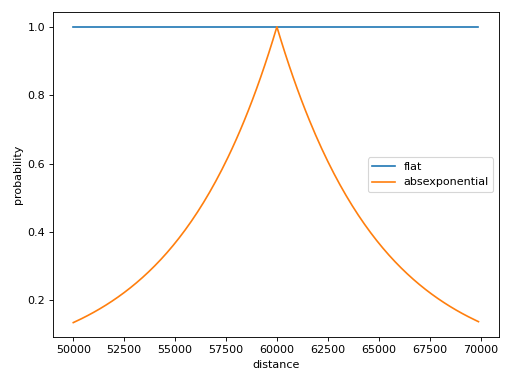## Extinction¶

### A(V)¶

The A(V) prior can be:

1. Flat

```av_prior_model = {'name': 'flat'}
```

2. Lognormal with the maximum at the A(V) given by max_pos and the width given by sigma.

```av_prior_model = {'name': 'lognormal',
'max_pos': 2.0,
'sigma': 1.0}
```
1. Two lognormals (see above for definition of terms)

```av_prior_model = {'name': 'two_lognormal',
'max_pos1': 0.2,
'max_pos2': 2.0,
'sigma1': 1.0,
'sigma2': 0.2,
'N1_to_N2': 1.0 / 5.0}
```
1. Exponential with decay rate ‘a’

```av_prior_model = {'name': 'exponential',
'a': 1.0}
```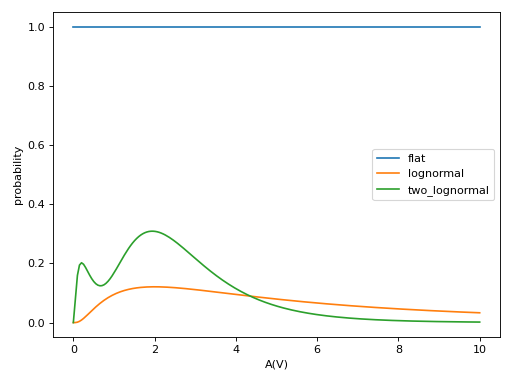### R(V)¶

1. Flat

```rv_prior_model = {'name': 'flat'}
```

2. Lognormal with the maximum at the R(V) given by max_pos and the width given by sigma.

```rv_prior_model = {'name': 'lognormal',
'max_pos': 3.1,
'sigma': 0.25}
```
1. Two lognormals (see above for definition of terms)

```rv_prior_model = {'name': 'two_lognormal',
'max_pos1': 3.1,
'max_pos1': 4.5,
'sigma1': 0.1,
'sigma2': 0.2,
'N1_to_N2': 2.0 / 5.0}
```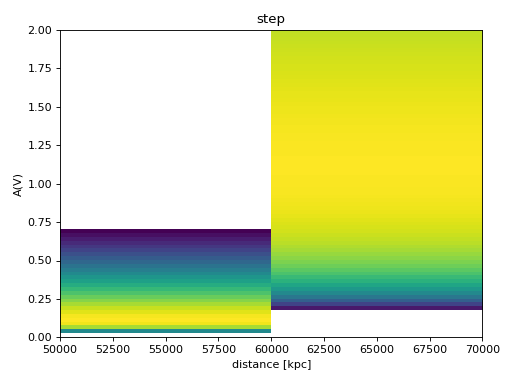### f_A¶

1. Flat

```fA_prior_model = {'name': 'flat'}
```

2. Lognormal with the maximum at the f_A given by max_pos and the width given by sigma.

```fA_prior_model = {'name': 'lognormal',
'max_pos': 0.8,
'sigma': 0.1}
```
1. Two lognormals (see above for definition of terms)

```fA_prior_model = {'name': 'two_lognormal',
'max_pos1': 0.1,
'max_pos1': 0.8,
'sigma1': 0.1,
'sigma2': 0.2,
'N1_to_N2': 2.0 / 5.0}
```